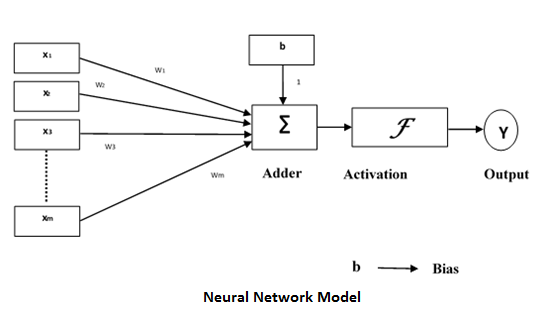# Fuzziness in Neural Networks

Artificial neural network (ANN) is a network of efficient computing systems the central theme of which is borrowed from the analogy of biological neural networks. ANNs are also named as “artificial neural systems,” parallel distributed processing systems,” “connectionist systems.” ANN acquires large collection of units that are interconnected in some pattern to allow communications between units. These units, also referred to as nodes or neurons, are simple processors which operate in parallel.

Every neuron is connected with other neuron through a connection link. Each connection link is associated with a weight having the information about the input signal. This is the most useful information for neurons to solve a particular problem because the weight usually inhibits the signal that is being communicated. Each neuron is having its internal state which is called the activation signal. Output signals, which are produced after combining the input signals and the activation rule, may be sent to other units. It also consists of a bias ‘b’ whose weight is always 1.## Why to use Fuzzy Logic in Neural Network

As we have discussed above that every neuron in ANN is connected with other neuron through a connection link and that link is associated with a weight having the information about the input signal. Hence we can say that weights have the useful information about input to solve the problems.

Following are some reasons to use fuzzy logic in neural networks −

• Fuzzy logic is largely used to define the weights, from fuzzy sets, in neural networks.

• When crisp values are not possible to apply, then fuzzy values are used.

• We have already studied that training and learning help neural networks perform better in unexpected situations. At that time fuzzy values would be more applicable than crisp values.

• When we use fuzzy logic in neural networks then the values must not be crisp and the processing can be done in parallel.

### Fuzzy Cognitive Map

It is a form of fuzziness in neural networks. Basically FCM is like a dynamic state machine with fuzzy states (not just 1 or 0).

### Difficulty in using Fuzzy Logic in Neural Networks

Despite having numerous advantages, there is also some difficulty while using fuzzy logic in neural networks. The difficulty is related with membership rules, the need to build fuzzy system, because it is sometimes complicated to deduce it with the given set of complex data.

## Neural-Trained Fuzzy Logic

The reverse relationship between neural network and fuzzy logic, i.e., neural network used to train fuzzy logic is also a good area of study. Following are two major reasons to build neuraltrained fuzzy logic −

• New patterns of data can be learned easily with the help of neural networks hence, it can be used to preprocess data in fuzzy systems.

• Neural network, because of its capability to learn new relationship with new input data, can be used to refine fuzzy rules to create fuzzy adaptive system.

## Examples of Neural-Trained Fuzzy system

Neural-Trained Fuzzy systems are being used in many commercial applications. Let us now see a few examples where Neural-Trained Fuzzy system is applied −

• The Laboratory for International Fuzzy Engineering Research (LIFE) in Yokohama, Japan has a back-propagation neural network that derives fuzzy rules. This system has been successfully applied to foreign-exchange trade system with approximately 5000 fuzzy rules.

• Ford Motor Company has developed trainable fuzzy systems for automobile idle-speed control.

• NeuFuz, software product of National Semiconductor Corporation, supports the generation of fuzzy rules with a neural network for control applications.

• AEG Corporation of Germany uses neural-trained fuzzy control system for its water – and energy conserving machine. It is having total of 157 fuzzy rules.